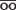# joule

joule
A unit of energy; the heat generated, or energy expended, by an ampere flowing through an ohm for 1 sec; equal to 107 ergs and to a newton-meter. It is an approved multiple of the SI fundamental unit of energy, the erg, and is intended to replace the calorie (4.184 J). SYN: unit of heat (3). [J.P. J.]

* * *

joule 'jü(ə)l n a unit of work or energy equal to the work done by a force of one newton acting through a distance of one meter
Joule James Prescott (1818-1889)
British physicist. Joule is famous for his experiments on heat. In a series of investigations he demonstrated that heat is a form of energy (regardless of the substance that is heated) and later determined quantitatively the amount of mechanical and electrical energy expended in the generation of heat energy. In 1843 he published his value for the amount of work required to produce a unit of heat, now known as the mechanical equivalent of heat. He established the principle that various forms of energy-mechanical, electrical, and heat-are basically the same and are interchangeable. This principle forms the basis of conservation of energy. The joule unit of work or energy was named in his honor in 1882.

* * *

n.
the SI units of work or energy, equal to the work done when the point of application of a force of 1 newton is displaced through a distance of 1 metre in the direction of the force. In electrical terms the joule is the work done per second when a current of 1 ampere flows through a resistance of 1 ohm. Symbol: J. See also calorie.

* * *

(J) (jl) [James Prescott Joule, English physicist, 1818â€“1889] the SI unit of quantity of energy, being the work done by a force of 1 newton acting over a distance of 1 meter in the direction of the force.

Medical dictionary. 2011.

### Look at other dictionaries:

• joule — joule …   Dictionnaire des rimes

• joule — [ ʒul ] n. m. • 1882; nom d un physicien ♦ Phys. Unité de mesure de travail, d énergie et de quantité de chaleur (symb. J), correspondant au travail d une force d un newton se déplaçant d un mètre dans la direction de la force. Une calorie vaut… …   Encyclopédie Universelle

• Joule — [dʒ̮u:l], das; [s], : Maßeinheit für die Energie (z. B. des Energieumsatzes des menschlichen Körpers; 1 Kalorie = 4,186 Joule): ein Glas Vollmilch hat 167 Joule. * * * Joule 〈[dʒaʊl] od. [ʒu:l] n.; s, ; 〉 Maßeinheit, SI Einheit der Energie,… …   Universal-Lexikon

• JOULE (J. P.) — Joule compte parmi les plus importants fondateurs de la thermodynamique. Parvenu en 1843 à donner une formulation stricte du principe de la conservation de l’énergie, il entreprit de quantifier les relations entre chaleur et travail au moyen… …   Encyclopédie Universelle

• joule — JOULE, jouli, s.m. Unitate de măsură a energiei, egală cu lucrul mecanic efectuat de forţa de un newton, când punctul ei de aplicaţie se deplasează cu un metru în direcţia şi în sensul forţei. [pr.: jul] – Din fr., enlg. joule. Trimis de cata, 13 …   Dicționar Român

• joule — (j[=oo]l), n. [From the distinguished English physicist, James Prescott Joule (1818 1889).] (Physics.) A unit of work which is equal to 10^{7} ergs (the unit of work in the C. G. S. system of units), and is equivalent to one watt second, the… …   The Collaborative International Dictionary of English

• Joule — se refiere a: Julio o joule (con símbolo J), unidad del Sistema Internacional para energía y trabajo. James Prescott Joule, físico inglés, en honor al cual se denominó la unidad anterior. El efecto Joule, relativo a la energía que disipa un… …   Wikipedia Español

• joule — [dʒuːl ǁ dʒuːl, dʒaʊl] written abbreviation J noun [countable] a unit of energy, work done, or quantity of heat * * * joule UK US /dʒuːl/ noun [C] (WRITTEN ABBREVIATION J) MEASURES ► …   Financial and business terms

• Joule — [von DIN u. anderen Organisationen festgelegte Aussprache nur dʒu:l, sonst auch dʒaul] das; [s], <nach dem engl. Physiker James Prescott Joule, 1818 1889> Maßeinheit für die Energie (z. B. den Energieumsatz des Körpers; 1 cal = 4,186… …   Das große Fremdwörterbuch

• Joule — 〈 [dʒaʊl] od. [ʒu:l] n.; Gen.: od. s, Pl.: ; Zeichen: J〉 Maßeinheit der Energie, 1 J = 1 Newtonmeter (Nm) = 1 Wattsekunde (Ws) = 0,102 Kilopondmeter (kpm) = 0,238 Kalorien (cal) = 1 m2kg/s2 [Etym.: nach dem engl. Physiker James Prescott Joule,… …   Lexikalische Deutsches Wörterbuch

• Joule — [nach DIN: ds̶c̶h̶u̱l, sonst auch: ds̶c̶h̶a̲u̲l; nach dem engl. Physiker J.JouleP. Joule, 1818 1889] s; [s], : Maßeinheit der Arbeit, Energie und Wärmemenge (z. B. für den Energiewert der aufgenommenen Nahrung; dafür bisher ↑Kalorie); Zeichen J… …   Das Wörterbuch medizinischer Fachausdrücke